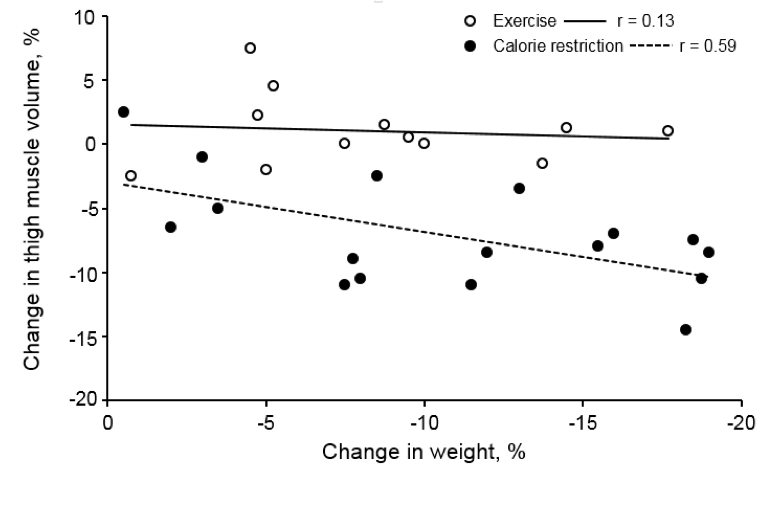## Information

A graph of data, whether it is a correlational graph or not, always tells some kind of story about the data being plotted. How do you figure out what that story is for a particular graph? What is it telling you and how is it telling it?

For any graph, you need to figure out the following:

1. What is being plotted?
2. Why is it being plotted?
3. What can I conclude from the data and its relationships?
4. What can I not yet conclude from the data?

If you are interpreting a correlational graph, you additionally need to ask yourself:

1. Why is the relationship between these data being highlighted?
2. How does the strength of the correlation (as revealed by the r or r2 value) affect my conclusions about the data and their relationship?
3. If there is more than one set of data being plotted, what does comparing their correlation coefficients allow me to conclude?

Remember, sometimes researchers plot data to show there is a correlation between them (and therefore, possible one is causing the other), and sometimes research plot data to show there is no correlation between them (and therefore, the one is having no effect on the other.)

### Lab 2 Exercises 2.7

Use the following graph as an example to walk through the steps of figuring out how to interpret a graph.Figure 2-5. Data from a journal article by a research group at the University of Washington School of Medicine (Weiss, et al. 2007. Lower extremity muscle size and strength and aerobic capacity decrease with caloric restriction but not with exercise-induced weight loss. J Appl Physiol 102: 634–640.)

The researchers were comparing weight loss from dieting (“caloric restriction”) to weight loss from exercise. They had two groups, each consisting of 18 men and women in their fifties. The first group were called the caloric restriction group and were put on a strict diet for three months. The second group were called the exercise group and were put on an exercise regimen for three months. After the three months, the researchers measured various indicators of strength in their test subjects. The researchers were interested if they could find a way to allow elderly people to lose weight without losing strength. This graph is the results of one of the researchers’ set of measurements.

1. Examine the graph. What does each individual data point represent?
2. What is being measured on the X axis and what aspect of the study is it reporting?
3. What is being measured on the Y axis and how is it an indicator of strength?
4. Why are the researchers interested in the correlations between these two variables in both the exercise-only group and the diet-only group?
5. The best-fit line through the exercise data (EX) has a value of r = 0.13. What does tell you about the relationship between exercising-induced weight loss and the changes in the size of the subjects’ thigh?
6. Why doesn’t the best-fit line through the exercise data (EX) have a value of r = 0.0?
7. The best-fit line through the dieting data (CR) has a value of r=0.59. This is a positive correlation. What does this tell you about the relationship between dieting-induced weight loss and changes in the size of the subjects’ thighs?
8. Why doesn’t the best-fit line though the dieting data (CR) have a value of r = 1.0?
9. This graph only tells us definitive information about how weight loss via exercise or dieting effect the size of the thigh muscles in these volunteers. But we can formulate more general hypotheses about the effect of dieting-induced weight loss vs. exercise-induced weight loss on overall strength based on these results. These more general hypotheses are not definitively supported yet, but they are suggested by these results. What are these more general hypotheses?